Saturday, July 11, 2020
Home > CBSE Class 12 > NCERT Solutions for Class 12 Maths Vector Algebra Exercise 10.3

# NCERT Solutions for Class 12 Maths Vector Algebra Exercise 10.3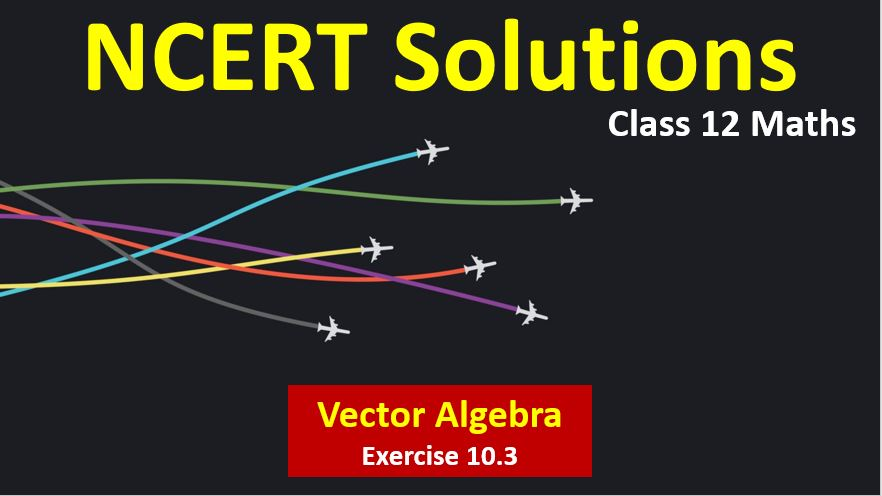# NCERT Solutions for Class 12 Maths Vector Algebra

Hi Students, Welcome to Amans Maths Blogs (AMB). In this post, you will get the NCERT Solutions for Class 12 Maths Vector Algebra Exercise 10.3.

As we know that all the schools affiliated from CBSE follow the NCERT books for all subjects. You can check the CBSE NCERT Syllabus. Thus, NCERT Solutions helps the students to solve the exercise questions as given in NCERT Books.

NCERT Solutions for class 12 is highly recommended by the experienced teacher for students who are going to appear in CBSE Class 12 and JEE Mains and Advanced and NEET level exams. Here You will get NCERT Solutions for Class 12 Maths Vector Algebra Exercise 10.3 of all questions given in NCERT textbooks of class 12 in details with step by step process.

The PDF books of NCERT Solutions for Class 12 are the first step towards the learning and understanding the each sections of Maths, Physics, Chemistry, Biology as it all help in engineering medical entrance exams. To solve it, you just need to click on download links of NCERT solutions for class 12.

CBSE Class 12th is an important school class in your life as you take some serious decision about your career. And out of all subjects, Maths is an important and core subjects. So CBSE NCERT Solutions for Class 12th Maths is major role in your exam preparation as it has detailed chapter wise solutions for all exercise. This NCERT Solutions can be downloaded in PDF file. The downloading link is given at last.

NCERT Solutions for Class 12 Maths are not only the solutions of Maths exercise but it builds your foundation of other important subjects. Getting knowledge of depth concept of CBSE Class 12th Maths like Algebra, Calculus, Trigonometry, Coordinate Geometry help you to understand the concept of Physics and Physical Chemistry.

Note: In this solution, the vector is represented by BOLD font. For example: a, b, OP, AB, i, j, k represent the vectors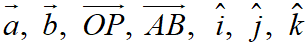## NCERT Solutions for Class 12 Maths Vector Algebra

NCERT Solutions for Class 12 Maths Vector Algebra Exercise 10.3: Ques No 1.

Find the angle between two vectors a and b with magnitudes √3 and 2 respectively having a.b = √6.

NCERT Solutions:

Given that |a| = √3 and |b| = 2 and a.b = √6.

Then, the angle between a and b is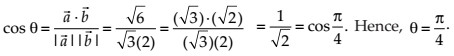NCERT Solutions for Class 12 Maths Vector Algebra Exercise 10.3: Ques No 2.

Find the angle between the vectors i – 2j + 3k and 3i – 2j + k.

NCERT Solutions:

Given that a = i – 2j + 3k and b = 3i – 2j + k.

Then,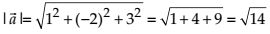a.b = (i – 2j + 3k).(3i – 2j + k) = 3 + 4 + 3 = 10

Then, the angle between the given vectors a and b is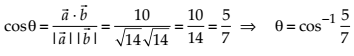NCERT Solutions for Class 12 Maths Vector Algebra Exercise 10.3: Ques No 3.

Find the projection of the vector ij on the vector i + j.

NCERT Solutions:

Given that a = ij and b = i + j.

Then, |b| =a.b = (ij).(i + j) = 1 – 1 = 0.

Thus, the projection of a on b isNCERT Solutions for Class 12 Maths Vector Algebra Exercise 10.3: Ques No 4.

Find the projection of the vector i + 3j + 7k on the vector 7ij + 8k.

NCERT Solutions:

Given that a = i + 3j + 7k and b = 7ij + 8k.

Then, |b| =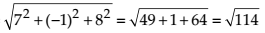a.b = (i + 3j + 7k).(7ij + 8k) = 7 – 3 + 56 = 60.

Thus, the projection of a on b is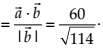NCERT Solutions for Class 12 Maths Vector Algebra Exercise 10.3: Ques No 5.

Show that each of the given three vectors is a unit vector:Also, show that they are mutually perpendicular to each other.

NCERT Solutions:

Given that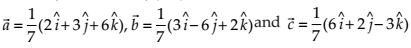.Thus, these vectors a, b and c are unit vectors.

Now,Hence, a and b are perpendicular vectors.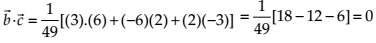Hence, b and c are perpendicular vectors.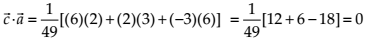Hence, c and a are perpendicular vectors.

Therefore, the vectors a, b and c are mutually vectors.

NCERT Solutions for Class 12 Maths Vector Algebra Exercise 10.3: Ques No 6.

Find |a| and |b|, if (a + b).(ab) = 8 and |a| = 8|b|.

NCERT Solutions: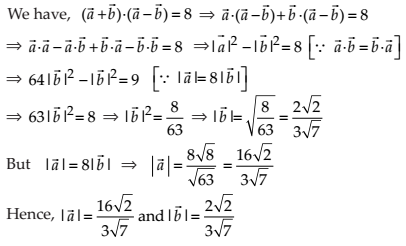NCERT Solutions for Class 12 Maths Vector Algebra Exercise 10.3: Ques No 7.

Evaluate the product (3a – 5b).(2a + 7b).

NCERT Solutions: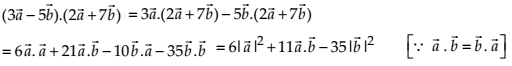NCERT Solutions for Class 12 Maths Vector Algebra Exercise 10.3: Ques No 8.

Find the magnitude of two vectors a and b, having the same magnitude and such that the angle between them is 60o and their scalar product is 1/2.

NCERT Solutions:

Given that |a| = |b|, θ = 60o and a.b = 1/2.NCERT Solutions for Class 12 Maths Vector Algebra Exercise 10.3: Ques No 9.

Find |x|, if for a unit vector a, (xa).(x + a) = 12.

NCERT Solutions:

Given that |a| = 1

(xa).(x + a) = 12.

⇒ |x|2 – |a|2 = 12

⇒ |x|2 – (1)2 = 12

⇒ |x|2 – 1 = 12

⇒ |x|2 = 13

⇒ |x| = √13

NCERT Solutions for Class 12 Maths Vector Algebra Exercise 10.3: Ques No 10.

If a = 2i + 2j + 3k, b = –i + 2j + k and c = 3i + j are such that a + λb is perpendicular to c, then find the value of λ.

NCERT Solutions: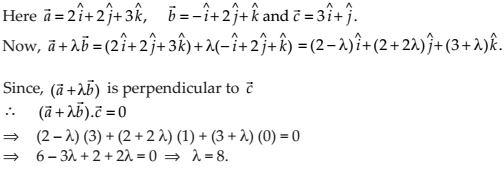NCERT Solutions for Class 12 Maths Vector Algebra Exercise 10.3: Ques No 11.

Show that |a|b + |b|a is perpendicular to |a|b – |b|a, for any two nonzero vectors a and b.

NCERT Solutions:NCERT Solutions for Class 12 Maths Vector Algebra Exercise 10.3: Ques No 12.

If aa = 0 and ab = 0, then what can be concluded about the vector b

NCERT Solutions:

Given that aa = 0 and ab = 0. It is clear that a = 0 (null vector) and b is any vector.

NCERT Solutions for Class 12 Maths Vector Algebra Exercise 10.3: Ques No 13.

If a, b, c are unit vectors such that a + b + c = 0, find the value of a.b + b.c + c.a.

NCERT Solutions:

Given that |a| = |b| = |c| = 1 and

a + b + c = 0

⇒ (a + b + c)2 = 0

⇒ |a|2 + |b|2 + |c|2 + 2(a.b + b.c + c.a) =  0

⇒ (1)2 + (1)2 + (1)2 + 2(a.b + b.c + c.a) =  0

⇒ 3 + 2(a.b + b.c + c.a) =  0

(a.b + b.c + c.a) = – 3/2.

NCERT Solutions for Class 12 Maths Vector Algebra Exercise 10.3: Ques No 14.

If either vector a = 0 or b = 0, then a.b = 0. But the converse need not be true. Justify your answer with an example.

NCERT Solutions:NCERT Solutions for Class 12 Maths Vector Algebra Exercise 10.3: Ques No 15.

If the vertices A, B, C of a triangle ABC are (1, 2, 3), (–1, 0, 0), (0, 1, 2), respectively, then find ∠ABC. [∠ABC is the angle between the vectors BA and BC].

NCERT Solutions: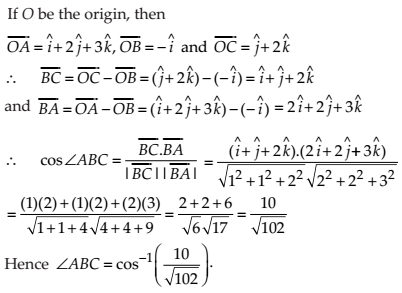NCERT Solutions for Class 12 Maths Vector Algebra Exercise 10.3: Ques No 16.

Show that the points A(1, 2, 7), B(2, 6, 3) and C(3, 10, –1) are collinear.

NCERT Solutions:NCERT Solutions for Class 12 Maths Vector Algebra Exercise 10.3: Ques No 17.

Show that the vectors 2ij + k, i − 3j − 5k and 3i − 4j − 4k form the vertices of a right angled triangle.

NCERT Solutions: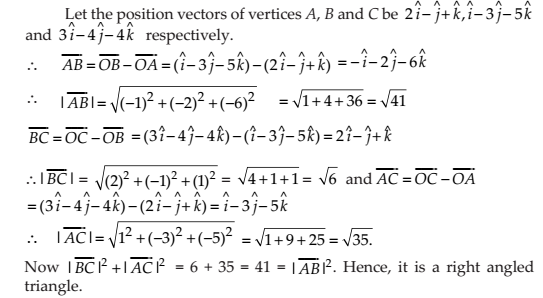NCERT Solutions for Class 12 Maths Vector Algebra Exercise 10.3: Ques No 18.

If a is a nonzero vector of magnitude ‘a’ and λ a nonzero scalar, then λa is unit vector if

(A) λ = 1

(B) λ = – 1

(C) a = |λ|

(D) a = 1/|λ|

NCERT Solutions:

(D) a is a non-zero vector of magnitude a. |a| = a

Since λa is a unit vector, then |λa| = 1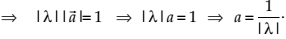error: Content is protected !!